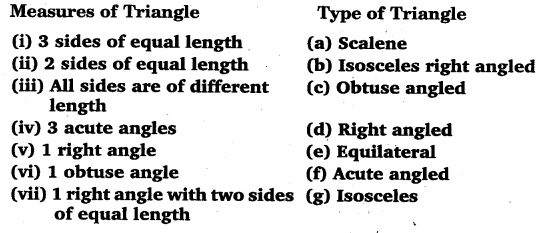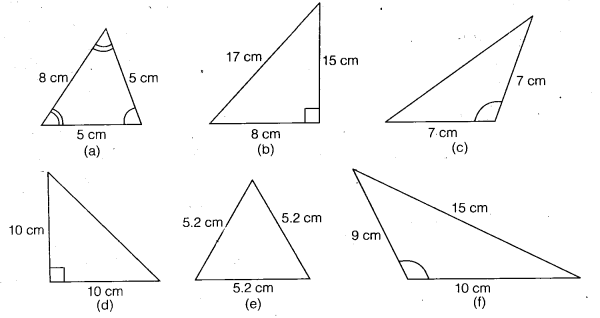# Class 6 Maths NCERT Solutions for Chapter 5 Understanding Elementary Shapes Ex – 5.6

## Understanding Elementary Shapes

Question 1.
Name the types of following triangles:

(a) Triangle with lengths of sides 7 cm, 8 cm and 9 cm.
(b) ∆AJBC with AB = 8.7 cm, AC = 7 cm and BC = 6 cm.
(c) ∆PQR such that PQ = QR = PR = 5 cm.
(d) ∆DEF with m∠D = 90°
(e) ∆XYZ with m∠Y = 90° and XY = YZ.
(f) ∆LMN with m∠L = 30°, m∠M = 70° and m∠N = 80°

Solution:
(a) Since all the sides are of different lengths
∴ It is scalene triangle.
(b) In ∆ABC, AB ≠ BC ≠ CA.
∴ AABC is scalene triangle.
(c) In ∆PQR, PQ = QR = PR
∴ ∆PQR is equilateral triangle.
(d) In ∆DEF, m∠D =90°
∴ ∆DEF is right angled triangle.
(e) In ∆ XYZ, m∠Y = 90° and XY = YZ
∴ ∆ XYZ is an isosceles right angled.
(f) As each angle of ∆ LMN is < 90°
∴ ∆ LMN is an acute angled triangle.

Question 2.
Match the following:Solution:
The matching is as shown:
(i) → (e)
(ii)→ (g)
(iii) → (a)
(iv) → (f)
(v) → (d)
(vi) → (c)
(vii) → (b)

Question 3.
Name each of the following triangles in two different ways: (you may judge the nature of the angle by observation)Solution:
(a)
Isosceles triangle; Acute angled triangle.
(b) Scalene triangle; Right angled triangle.
(c) Isosceles triangle; Obtuse angled triangle.
(d) Isosceles triangle; Right angled triangle.
(e) Equilateral triangle; Acute angled triangle.
(f) Scalene triangle; Obtuse angled triangle.

Question 4.
Try to construct triangles match sticks. Some are shown here. Gan you make a triangle with

(a) 3 matchsticks?
(b) 4 matchsticks?
(c) 5 matchsticks?
(d) 6 matchsticks?
(Remember you have to use all the available matchsticks in each case)
Name the type of triangle in each case.
If you cannot make a triangle, think of reasons for it.Solution:
(a) Yes, an equilateral triangle.
(b) No (as sum of two sides is always > the third side but here 1 + 1 = 2 ≱ ≯ 2).
(c) Yes, an isosceles triangle.
(d) Yes, an equilateral triangle.